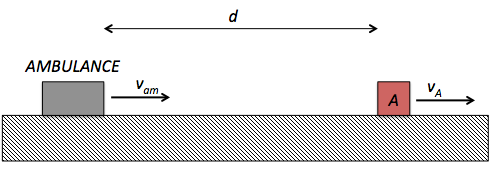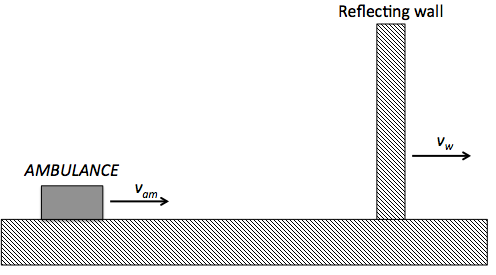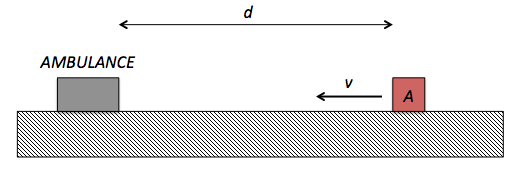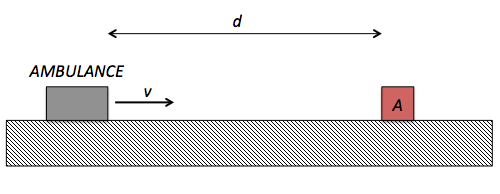Classical Mechanics

# Doppler EffectAs shown in the above diagram, person $A$ driving a car with speed $v_A = 17 \text{ m/s}$ hears a siren sound with frequency $f_ A = 737 \text{ Hz}$ at a distance of $d = 141 \text{ m}$ behind him, coming from an ambulance chasing his car with speed $v_{am} = 34 \text{ m/s}.$ What is the approximate frequency of the siren sound heard in the ambulance?

Assumption

• The speed of sound is $u = 340 \text{ m/s}.$An ambulance sounding a siren of frequency $f_{am} = 687 \text{ Hz}$ is traveling towards a vertical reflecting wall with a speed of $v_{am} = 15 \text{ m/s}.$ If the wall is moving in same direction as the ambulance with a speed of $v_w = 5 \text{ m/s},$ what is the approximate beat frequency heard by the driver of the ambulance?

Assumption

• The speed of sound is $u = 340 \text{ m/s}.$

A person is riding a merry-go-round which has a radius of $r = 3 \text{ m}$ and an angular speed of $\omega = 3 \text{ rad/s}.$ If the person starts to blow a whistle of frequency $f= 485 \text{ Hz},$ what is the approximate highest frequency heard by a listener at rest a long distance away?

Assumption

• The speed of sound is $u = 340 \text{ m/s}.$As shown in the above diagram, person $A$ drives a car with speed $v = 16 \text{ m/s}$ at a distance of $d = 119 \text{ m}$ away from an ambulance standing still and sounding a siren with frequency $f_ A = 763 \text{ Hz}.$ What is the approximate frequency of the siren sound heard in the car?

Assumption

• The speed of sound is $u = 340 \text{ m/s}.$As shown in the above diagram, person $A$ hears an ambulance which heads towards him at a speed of $v = 14 \text{ m/s}$ sounding a siren with frequency $f_A = 775 \text{ Hz}$ at a distance of $d = 104 \text{ m}$ away from him. What is the approximate frequency of the siren sound heard in the ambulance?

Assumption

• The speed of sound is $u = 340 \text{ m/s}.$
×